Energy Equation & Fourier's Law

# Energy Equation & Fourier's Law Notes | Study Heat Transfer - Mechanical Engineering

## Document Description: Energy Equation & Fourier's Law for Mechanical Engineering 2022 is part of Introduction to Heat Transfer for Heat Transfer preparation. The notes and questions for Energy Equation & Fourier's Law have been prepared according to the Mechanical Engineering exam syllabus. Information about Energy Equation & Fourier's Law covers topics like and Energy Equation & Fourier's Law Example, for Mechanical Engineering 2022 Exam. Find important definitions, questions, notes, meanings, examples, exercises and tests below for Energy Equation & Fourier's Law.

Introduction of Energy Equation & Fourier's Law in English is available as part of our Heat Transfer for Mechanical Engineering & Energy Equation & Fourier's Law in Hindi for Heat Transfer course. Download more important topics related with Introduction to Heat Transfer, notes, lectures and mock test series for Mechanical Engineering Exam by signing up for free. Mechanical Engineering: Energy Equation & Fourier's Law Notes | Study Heat Transfer - Mechanical Engineering
 1 Crore+ students have signed up on EduRev. Have you?

Fourier's Law

The constitutive equation for conduction, we have see, is Fourier's Law. It says that the heat flux vector is a linear function of the temperature gradient, that is :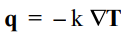What we mean by the notation is the following:

q = qi ei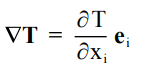Then for each of the components of q, we have the relation: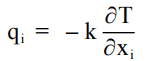Thermal Properties of Matter

Thermal conductivity

The conductivity is a material property that is a very strong function of the state of the material. The range of values goes from less than 0.01 Watts/m-°K for gaseous CO2 to over 600 Watts/m-°K for Ag metal. The change may be as much as 5 orders of magnitude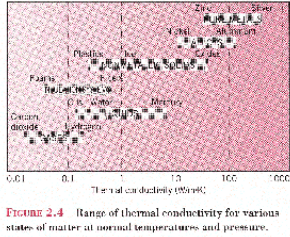Thermal Conductivity of Gases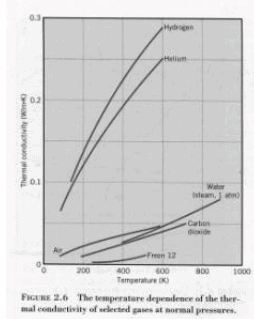Thermal Conductivity of Solids

The thermal conductivity of solids differ significantly as the next figure shows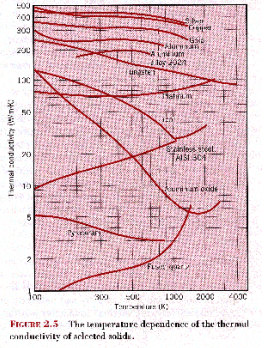Conductive Loss through a Window Pane

Examine the simple one-dimensional conduction problem as heat flow through a windowpane. The window glass thickness, L, is 1/8 in. If this is the only window in a room 9x12x8 or 864 ft3 , the area of the window is 2 ft x 3 ft or 6 ft2 .

Recall that qx is the heat flux and that k is the thermal conductivity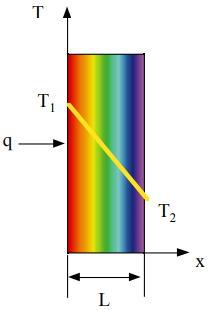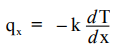The energy at steady state yielded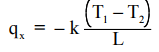The room is well heated and the temperature is uniform, so the heat low through the windowpane is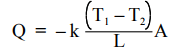If the room temperature is 60 °F and the exterior temperature is 20 °F, and k is 0.41 Btu/hr-ft2-°F then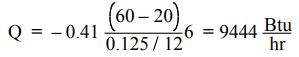Energy balance on the Room

How long does it take for the room temperature to change from 60 °F to 45 °F?

To make this estimate, we need to solve an energy balance on the room. A simple analysis yields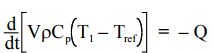Recognizing that heat capacity density are essentially constant, the equation becomes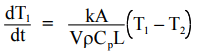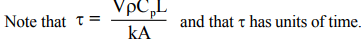At the outset, T1 = T10 = 60 °F The solution of the differential equation representing the energy balance is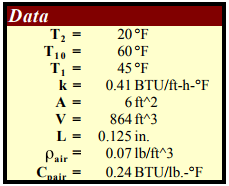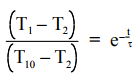To solve for the time required to get to 46 °F, we need all the data in the table.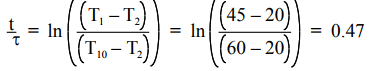It follows that τ = 0.47, τ = 1.75 minutes

Heat Conduction in a Composite Solid

Examine the simple one-dimensional conduction problem as heat flow through a thermally insulated windowpane. Each layer of window glass thickness, L, is 1/16 in. The insulation layer of air between the two panes is also 1/16 in. Recall that qx is the heat flux and that k is the thermal conductivity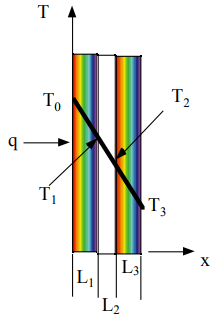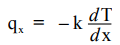The energy at steady state yielded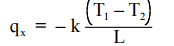The heat flow through the glass is given by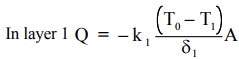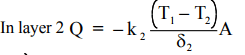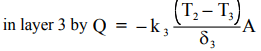Then we can rewrite the equations in this form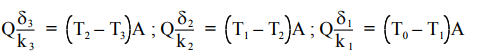If we add the three equations, we obtain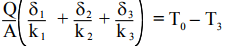We can consider the thickness/conductivity as a resistance so that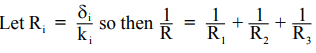The heat flow is then of the following form :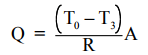This is like a problem of current flow in a series circuit. In the single pane problem discussed in Lecture 1, we noted that the resistance, δ/k, was 1/(192(0.41) = 0.254 hr-ft2 -°F/Btu. Recall that for the problem of cooling the room, t was 1.75 minutes. The thermal conductivity of air is 0.014 Btu/hr-ft-°F. so that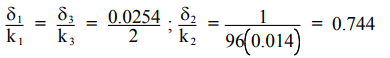as a consequence the reciprocal of the overall resistance is 0.744 + (0.0254) = 0.746.

Then we see that τ = (1 min) (0.746/0.0254) = 29.37 min

The Convective Boundary Condition

Again consider a windowpane, but now there is a heat transfer limitation at one boundary described by a boundary condition. qx = – h (Tr – Ti)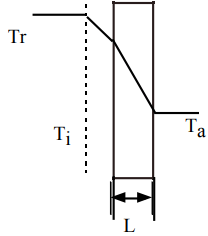Conduction through the glass is described by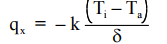The flux is constant at any cross-section so that we can write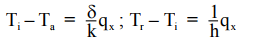Solving for the temperatures we get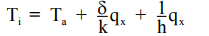Solving for qx , the relation becomes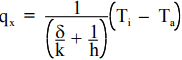Which modified shows a correction to the heat transfer coefficient modulated by the conduction problem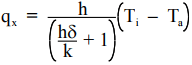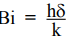The dimensionless number in the denominator is the Biot number, a ratio of the convective heat transfer coefficient to the equivalent heat transfer coefficient due to conduction.

Heat Transfer across a Composite Cylindrical Solid.

In the case of heat transfer in a cylinder, there is radial symmetry do that heat conduction is important only in the radial direction.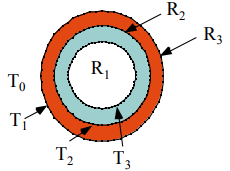The heat flux in the radial direction is given by Fourier’s law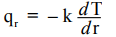The total heat flow through any circular surface is constant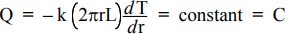Rearranging we obtain a relation for the temperature gradient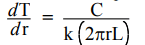which upon separation of variables is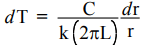An indefinite integration yields the temperature profile.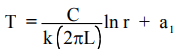The boundary conditions are

at r = R, T = T1 ;

at r = R2 , T = T2

at r = R3 , T = T3

qr2 = qr2

qr= h(T3 - T0 )

so that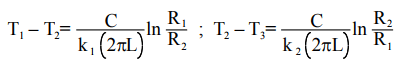It follows that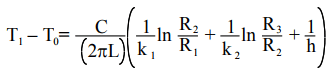This can be expressed as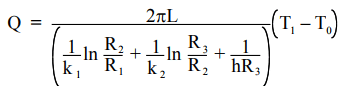Optimal Insulation on a Pipe

Is there an optimal thickness for the exterior insulation? In the context of the problem just formulated, is there a best value for R3?

Note that Q = f(R).

To find an extremum,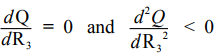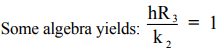It offers a critical radius for R= k2 /h beyond which the heat loss increases.

The document Energy Equation & Fourier's Law Notes | Study Heat Transfer - Mechanical Engineering is a part of the Mechanical Engineering Course Heat Transfer.
All you need of Mechanical Engineering at this link: Mechanical Engineering

## Heat Transfer

58 videos|70 docs|85 tests
 Use Code STAYHOME200 and get INR 200 additional OFF

## Heat Transfer

58 videos|70 docs|85 tests

Track your progress, build streaks, highlight & save important lessons and more!

,

,

,

,

,

,

,

,

,

,

,

,

,

,

,

,

,

,

,

,

,

;